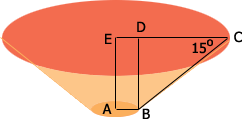SEARCH HOMEMath Central Quandaries & QueriesQuestion from Sameer: Bottom cone radius 1124.hight is 200. Top radius 15degree decrease to bottom radius. Top radius how much ?Hi,

Below is my sketch the situation you described. My sketch is not to scale. $AB$ is the radius of the base circle so $|AB| = 1124$ units. The height $|AE| = |BD| = 200$ units and the radius $|EC|$ of the top circle is unknown. I'm going to call it $R$ units. The measure of the angle $BCD$ is 15 degrees.The length of $ED$ is 1124 units so the length of $DC$ is $R - 1124$ units. The triangle $BCD$ is a right triangle and hence

$\tan(15^o) = \frac{|BD|}{|DC|} = \frac{200}{R-1124}.$

Thus

$R - 1124 = \frac{200}{\tan(15^o} = \frac{200}{0.2679} = 746.4.$

Hence

$R = 1124 + 746.4 = 1870.4 \mbox{ units.}$

Penny* Registered trade mark of Imperial Oil Limited. Used under license.Math Central is supported by the University of Regina and the Imperial Oil Foundation.